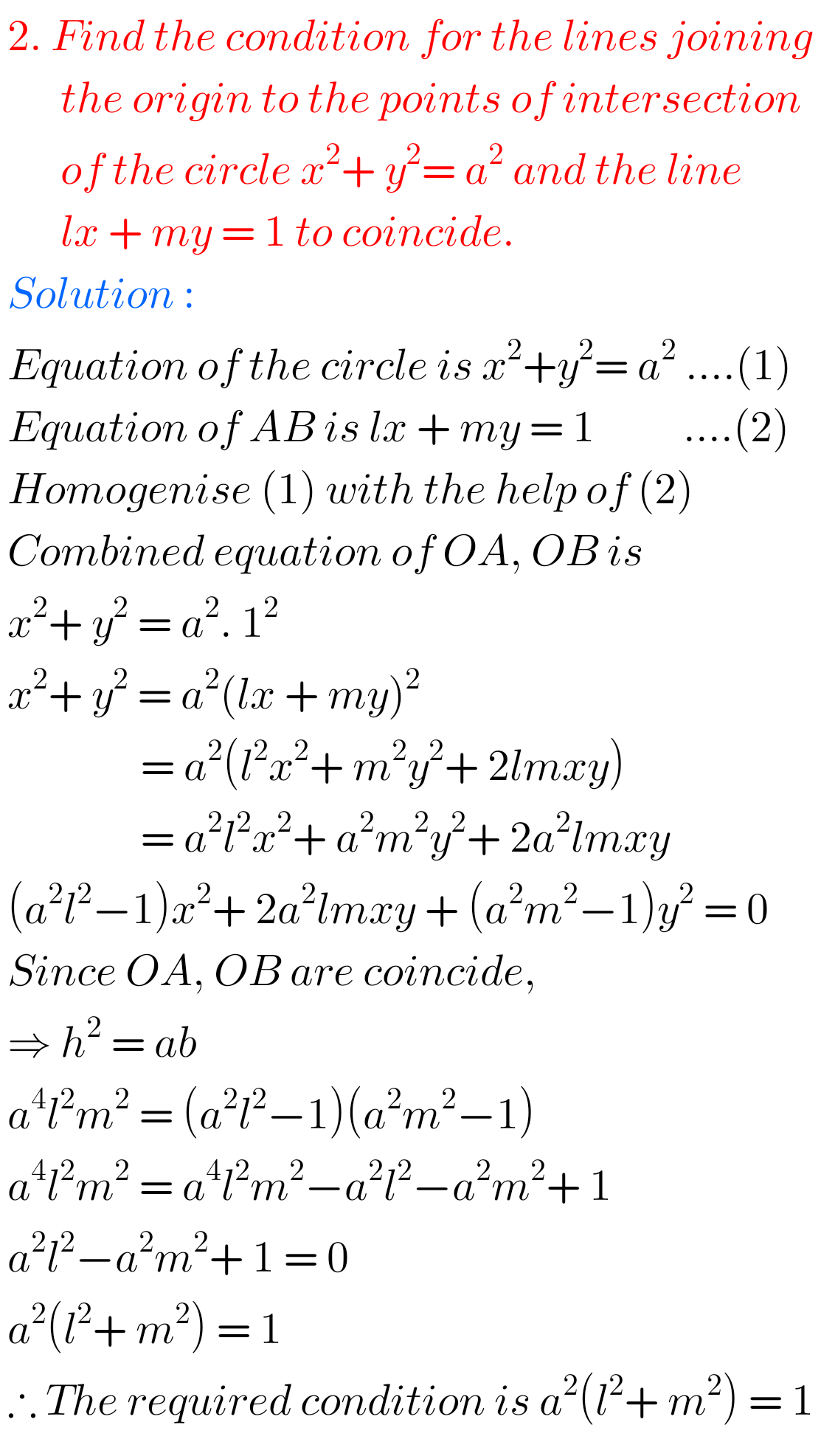## Pair Straight Lines exercise 4(c) solutions Inter Maths 1B

Intermediate Maths Solutions for Pair of Straight Lines Intermediate maths 1B Pair of Straight Lines exercise 4(b) solutions are given. Study the textbook lesson Pair of Straight Lines very well. Observe the example problems and solutions given in the textbook. Observe the given below solutions and try them in your own method. Inter Maths solutions …